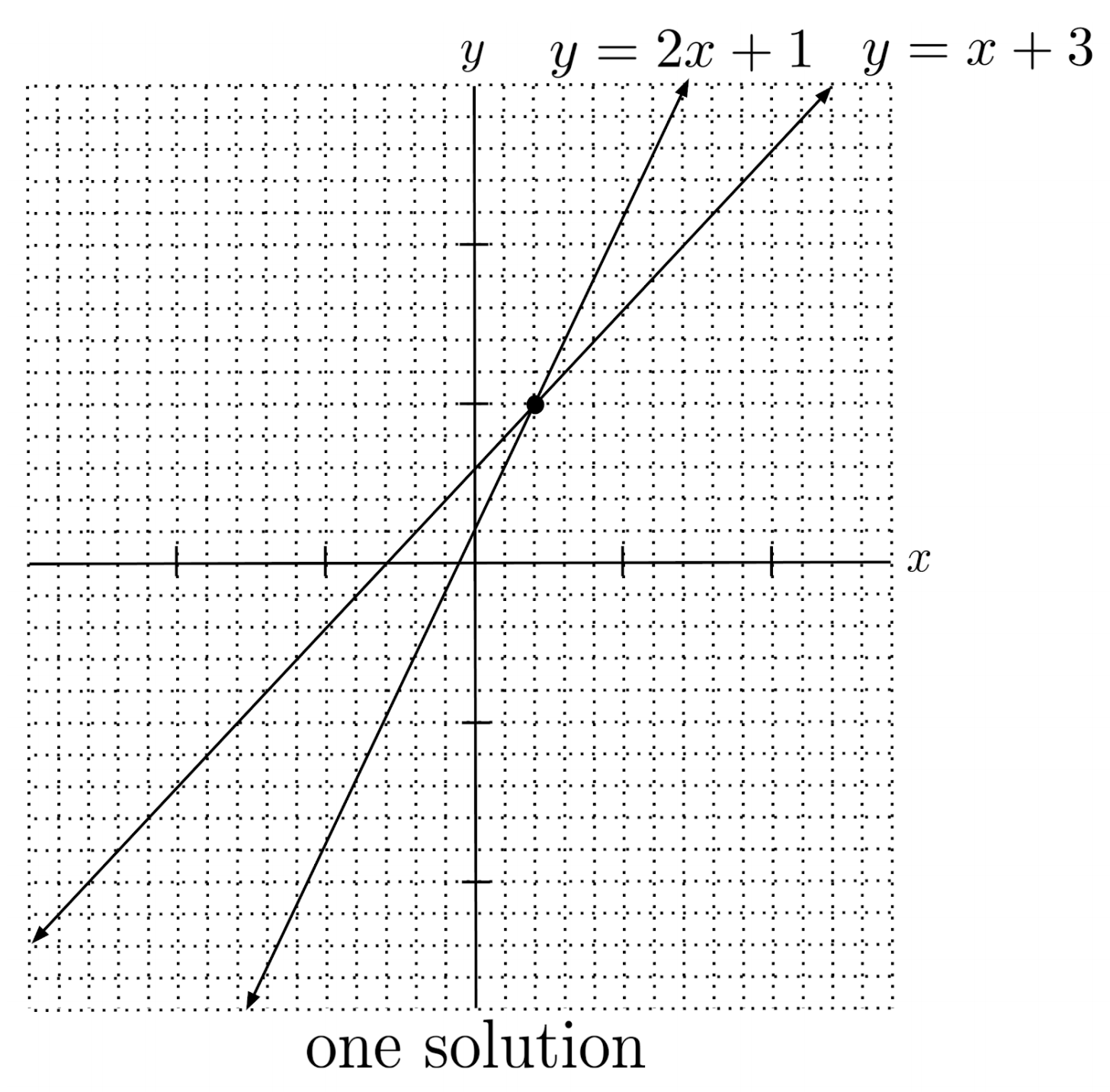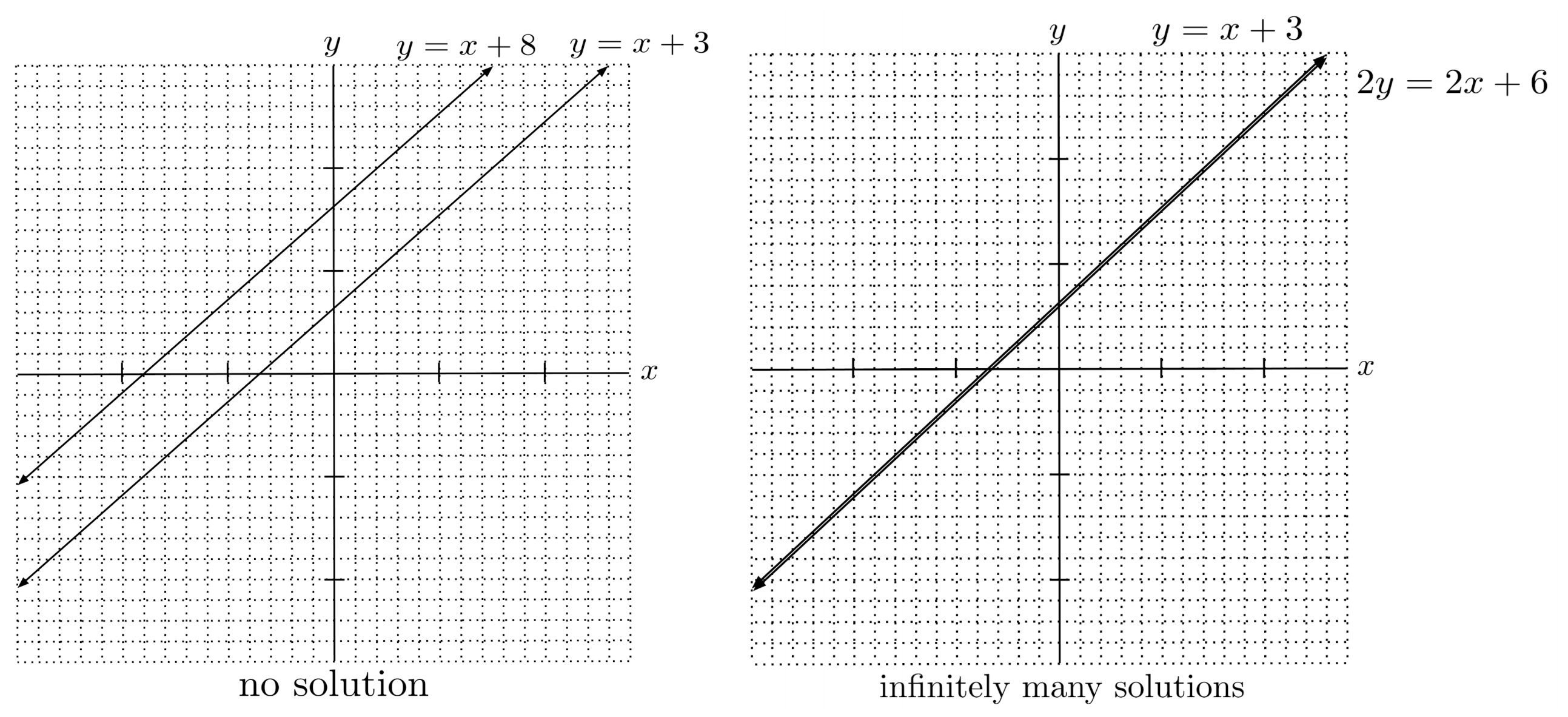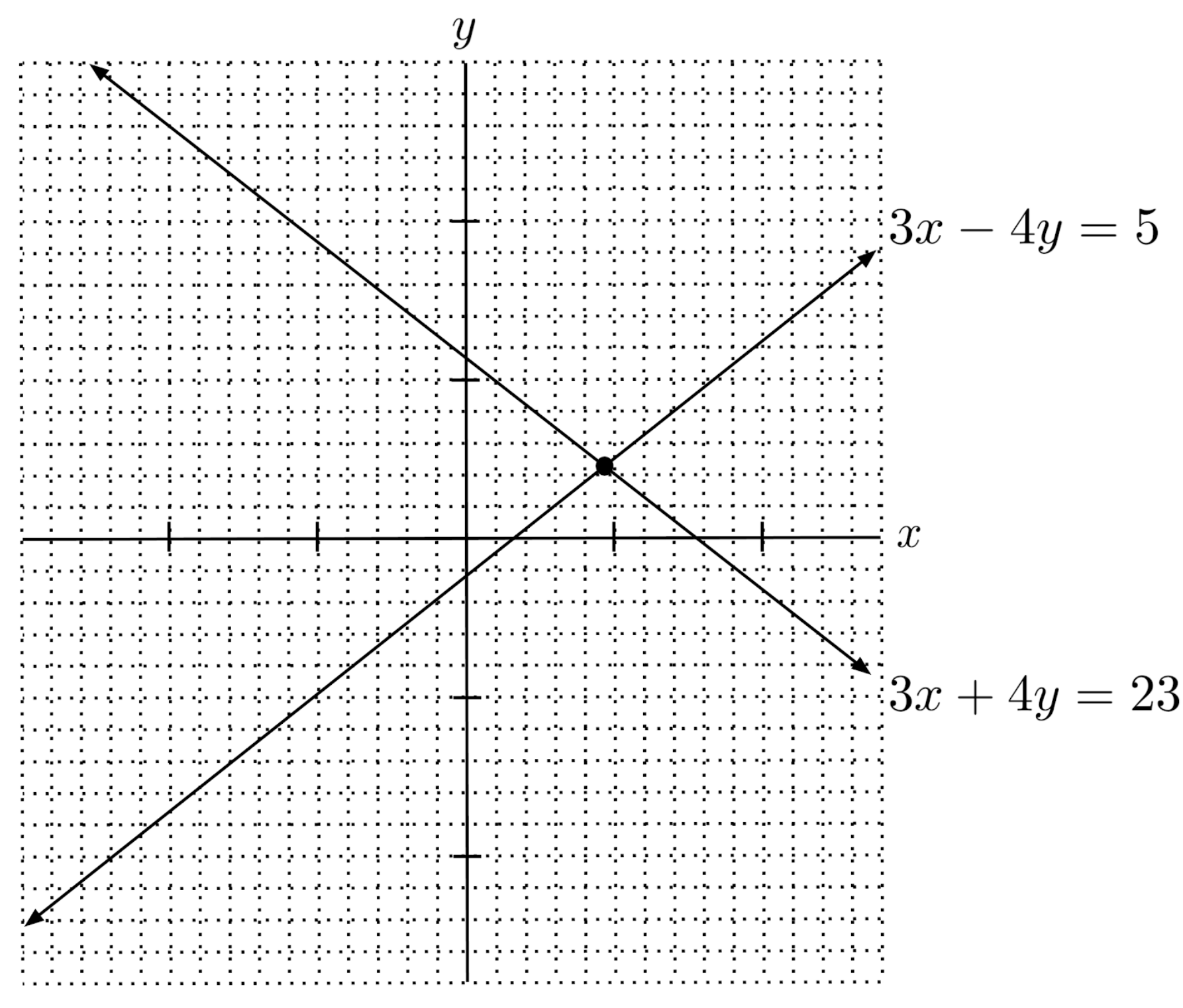# Linear Systems

A linear system consists of multiple linear equations, and the solution of a linear system consists of the pairs that satisfy all of the equations.

This post is part of a series.

A linear system consists of multiple linear equations, and the solution of a linear system consists of the pairs that satisfy all of the equations.

For example, the solution to the linear system

$\begin{cases} y=2x+1 \\ y=x+3 \end{cases}$

is $(2,5)$ because substituting $2$ for $x$ and $5$ for $y$ makes both equations true.

## Graphical Interpretation

Graphically, we can think of a linear system as being a set of two lines, and their solution as the point where they intersect.

The intersection point is the solution because it is on both lines, meaning it makes both equations true.Usually, two lines will intersect in exactly one point, and thus the system will have a single solution. However, when the two lines are parallel, meaning that they have the same slope, the lines will never intersect, unless they are actually the same line.

If the system consists of two different parallel lines, then it will have no solution because there are no intersection points. But if the system consists of two lines that are actually the same, then the system will have infinitely many solutions because every point on the line is a solution.We can sometimes tell the solution of a system by graphing the equations and looking for the point where they intersect. However, when the lines intersect at a point that doesn’t coincide with grid lines on the graph, it can be difficult to identify the exact coordinates of the intersection point.

For example, can you identify the point of intersection below? If you think you can, would you bet your life on it?## Substitution

There is another method for solving a system of linear equations, called the method of substitution, which makes it possible to solve a linear system without graphing it.

To perform substitution, we create a third equation by solving for a particular variable in the first and second equations and setting the results equal to each other.

Since the third equation has a single variable, we can solve for the numeric value of that variable, and then use it to find the numeric value of the other variable.

\begin{align*} \text{Given system} \hspace{.5cm} &\Bigg| \hspace{.5cm} \begin{cases} 3x+4y=23 \\ 3x-4y=5 \end{cases} \\ \text{Solve for } y \hspace{.5cm} &\Bigg| \hspace{.5cm} \begin{cases} 4y=-3x+23 \\ -4y=-3x+5 \end{cases} \\ \text{ } \hspace{.5cm} &\Bigg| \hspace{.5cm} \begin{cases} y=-\frac{3}{4} x + \frac{23}{4} \\ y = \frac{3}{4}x-\frac{5}{4} \end{cases} \\ \begin{matrix} \text{Set the results equal} \\ \text{to each other} \end{matrix} \hspace{.5cm} &\Bigg| \hspace{.5cm} -\frac{3}{4} x + \frac{23}{4} = \frac{3}{4}x-\frac{5}{4} \\ \text{Solve for } x \hspace{.5cm} &\Bigg| \hspace{.5cm} \frac{28}{4} = \frac{6}{4} x \\ \text{ } \hspace{.5cm} &\Bigg| \hspace{.5cm} x = \frac{14}{3} \\ \begin{matrix} \text{Substitute } x=\frac{14}{3} \text{ in} \\ \text{equation for } y \end{matrix} \hspace{.5cm} &\Bigg| \hspace{.5cm} y = \frac{3}{4} \left( \frac{14}{3} \right) - \frac{5}{4} \\ \text{ } \hspace{.5cm} &\Bigg| \hspace{.5cm} y = \frac{9}{4} \\ \text{Final solution} \hspace{.5cm} &\Bigg| \hspace{.5cm} \left( \frac{14}{3}, \frac{9}{4} \right) \end{align*}

To perform substitution even more quickly, instead of solving for a particular variable in both equations, we can solve for a particular variable in just one of the equations and then substitute the resulting expression where the particular variable occurs in the other equation.

\begin{align*} \text{Given system} \hspace{.5cm} &\Bigg| \hspace{.5cm} \begin{cases} 3x+4y=23 \\ 3x-4y=5 \end{cases} \\ \begin{matrix} \text{Solve for } y \text{ in bottom} \\ \text{equation} \end{matrix} \hspace{.5cm} &\Bigg| \hspace{.5cm} -4y = -3x+5 \\ \text{ } \hspace{.5cm} &\Bigg| \hspace{.5cm} y = \frac{3}{4}x - \frac{5}{4} \\ \text{Substitute into top equation} \hspace{.5cm} &\Bigg| \hspace{.5cm} 3x+4 \left( \frac{3}{4}x - \frac{5}{4} \right) = 23 \\ \text{Solve for } x \hspace{.5cm} &\Bigg| \hspace{.5cm} 3x+3x-5=23 \\ \text{ } \hspace{.5cm} &\Bigg| \hspace{.5cm} x = \frac{14}{3} \\ \begin{matrix} \text{Substitute } x=\frac{14}{3} \text{ in} \\ \text{equation for } y \end{matrix} \hspace{.5cm} &\Bigg| \hspace{.5cm} y = \frac{3}{4} \left( \frac{14}{3} \right) - \frac{5}{4} \\ \text{ } \hspace{.5cm} &\Bigg| \hspace{.5cm} y = \frac{9}{4} \\ \text{Final solution} \hspace{.5cm} &\Bigg| \hspace{.5cm} \left( \frac{14}{3}, \frac{9}{4} \right) \end{align*}

Remember that some systems have no solutions, and other solutions have infinite solutions – so it shouldn’t throw us off if the third equation created by substitution has no solutions or infinite solutions.

## Elimination

An even faster way to solve some linear equations is the method of elimination. The method of elimination also creates a third equation in a single variable, but it does so by adding multiples of the two original equations to cancel out one of the variables.

\begin{align*} \text{Given system} \hspace{.5cm} &\Bigg| \hspace{.5cm} \begin{cases} 3x+4y=23 \\ 3x-4y=5 \end{cases} \\ \text{Add the two equations} \hspace{.5cm} &\Bigg| \hspace{.5cm} 3x+3x+4y-4y=23+5 \\ y \text{ cancels} \hspace{.5cm} &\Bigg| \hspace{.5cm} 6x=28 \\ \text{Solve for } x \hspace{.5cm} &\Bigg| \hspace{.5cm} x = \frac{14}{3} \\ \begin{matrix} \text{Substitute } x=\frac{14}{3} \text{ in} \\ \text{top equation} \end{matrix} \hspace{.5cm} &\Bigg| \hspace{.5cm} 3 \left( \frac{14}{3} \right) + 4y = 23 \\ \text{Solve for } y \hspace{.5cm} &\Bigg| \hspace{.5cm} 14+4y=23 \\ \text{ } \hspace{.5cm} &\Bigg| \hspace{.5cm} y = \frac{9}{4} \\ \text{Final solution} \hspace{.5cm} &\Bigg| \hspace{.5cm} \left( \frac{14}{3}, \frac{9}{4} \right) \end{align*}

In the previous example, one of the variables cancelled when we added the two equations. Other times, though, no variable will cancel right away, and we will first need to multiply one of the equations by a number so that a variable will cancel when we add the equations.

\begin{align*} \text{Given system} \hspace{.5cm} &\Bigg| \hspace{.5cm} \begin{cases} x+2y=4 \\ 3x+4y=1 \end{cases} \\ \begin{matrix} \text{Multiply top equation} \\ \text{by } -2 \end{matrix} \hspace{.5cm} &\Bigg| \hspace{.5cm} \begin{cases} -2x-4y=-8 \\ 3x+4y=1 \end{cases} \\ \begin{matrix} \text{Add the two equations} \\ \text{to cancel } y \end{matrix} \hspace{.5cm} &\Bigg| \hspace{.5cm} x=-7 \end{align*}

Other times still, we may need to multiply both equations by a different number to cancel a variable. (We can just take the least common multiple – the same trick we use to add fractions with different denominators.)

\begin{align*} \text{Given system} \hspace{.5cm} &\Bigg| \hspace{.5cm} \begin{cases} 2x+3y=1 \\ 3x+5y=2 \end{cases} \\ \begin{matrix} \text{Multiply top equation by } -3 \\ \text{Multiply bottom equation by } 2 \\ \text{(Least common multiple is } 6 \text{)} \end{matrix} \hspace{.5cm} &\Bigg| \hspace{.5cm} \begin{cases} -6x-9y=-3 \\ 6x+10y=4 \end{cases} \\ \begin{matrix} \text{Add the two equations} \\ \text{to cancel } x \end{matrix} \hspace{.5cm} &\Bigg| \hspace{.5cm} y=1 \end{align*}

Again, since some systems have no solutions, and other solutions have infinite solutions, we should not be worried if the third equation created by elimination simplifies to a never-true statement like $2=1$ (no solutions) or an always-true statement like $1=1$ (infinite solutions).

## Exercises

Solve by substitution or elimination. (You can view the solution by clicking on the problem.)

$1) \hspace{.5cm} \begin{cases} y=2x-3 \\ y=x-1 \end{cases}$
Solution:
$\left( 2,1 \right)$

$2) \hspace{.5cm} \begin{cases} y=5x+1 \\ y=5x-1 \end{cases}$
Solution:
$\text{no solution}$

$3) \hspace{.5cm} \begin{cases} 2x+y=5 \\ x-y=4 \end{cases}$
Solution:
$\left( 3,-1 \right)$

$4) \hspace{.5cm} \begin{cases} 5x+2y=9 \\ 2x-y=-9 \end{cases}$
Solution:
$\left( -1,7 \right)$

$5) \hspace{.5cm} \begin{cases} 3x+4y=2 \\ 15x+20y=10 \end{cases}$
Solution:
$\text{infinitely many solutions}$

$6) \hspace{.5cm} \begin{cases} y+2x=3 \\ x+2y=3 \end{cases}$
Solution:
$\left( 1,1 \right)$

$7) \hspace{.5cm} \begin{cases} 5x-3y=4 \\ 40y+10x=31 \end{cases}$
Solution:
$\left( \frac{11}{10}, \frac{1}{2} \right)$

$8) \hspace{.5cm} \begin{cases} 10x=2y+3 \\ y=5x \end{cases}$
Solution:
$\text{no solution}$

$9) \hspace{.5cm} \begin{cases} 13=x+4y \\ x-y=5 \end{cases}$
Solution:
$\left( \frac{33}{5}, \frac{8}{5} \right)$

$10) \hspace{.5cm} \begin{cases} 5+2y=7x \\ x+3y=10 \end{cases}$
Solution:
$\left( \frac{35}{23}, \frac{65}{23} \right)$

This post is part of a series.